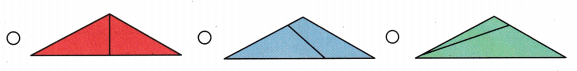Refer to our Texas Go Math Grade 1 Answer Key Pdf to score good marks in the exams. Test yourself by practicing the problems from Texas Go Math Grade 1 Lesson 16.1 Answer Key Equal or Unequal Parts.

## Texas Go Math Grade 1 Lesson 16.1 Answer Key Equal or Unequal Parts

Explore

Draw to show the parts.
Show 2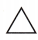Show 3FOR THE TEACHER • Have children draw lines to show two triangles in one square and three triangles in the other square.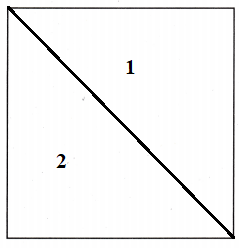The square is divided into two equal partsThe square is divided into two unequal parts

Math Talk
Mathematical Processes

Are all of the triangles the same? Explain.
Answer: No,all the triangles are not same some may be having equal sides and some might have unequal sides

Model and DrawShare and Show

Describe the parts. Write equal parts or unequal parts.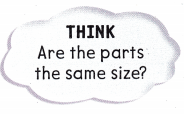Question 1.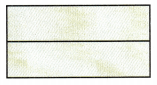Explanation:
The rectangle is divided at the center and is divided into equal halves

Question 2.Explanation:
The square is divided at the center and is divided into equal halves

Question 3.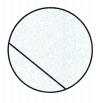Explanation:
The circle is divided into two unequal halves

Question 4.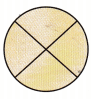Explanation:
The circle is divided into Four equal halves

Question 5.Explanation:
The square is divided into two unequal halves

Question 6.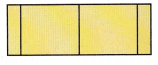Explanation:
The rectangle is divided into four unequal halves

Problem Solving

Color the shapes that show unequal shares.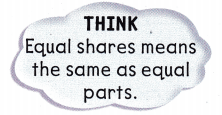Question 7.Color the shapes that show equal shares.

Question 8.H.O.T. Write the number of equal shares.

Question 9.__________ equal shares

Question 10.__________ equal shares

H.O.T. Multi-Step Draw lines to show the parts.

Question 11.
2 equal shares2 unequal shares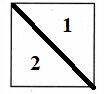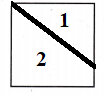Question 12.
4 equal shares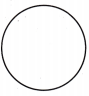4 unequal shares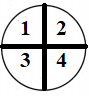Question 13.
Gordon has a wheel of cheese. He will share this cheese with Denny. Which wheel shows 2 equal parts?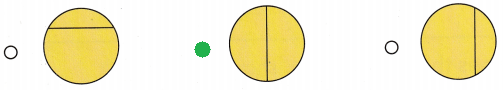Question 14.
Analyze Lena is cutting a square cake to have equal shares with 3 friends. Which square shows 4 equal parts?Question 15.
Multi-Step Henry has a loaf of bread shaped like a rectangle. He wants to cut it into two equal parts. Then he wants to cut each part into two more equal parts. How many parts will Henry have?(A) 2
(B) 6
(C) 4
Explanation:
henry first cuts the bread into 2 equal parts the he will have two parts
Later he cuts the both parts into 2 equal parts the the will have 2 breads with 2 equal halves that is 4 parts

Question 16.
Texas Test Prep Which shows equal shares?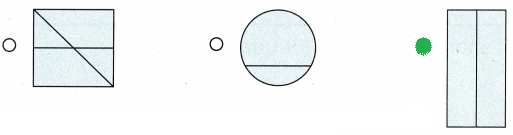TAKE HOME ACTIVITY • Draw a circle on a piece of paper. Ask your child to draw a line so the circle shows 2 equal shares.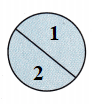### Texas Go Math Grade 1 Lesson 16.1 Homework and Practice Answer Key

Circle the shape that shows unequal parts.

Question 1.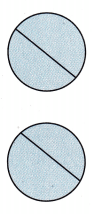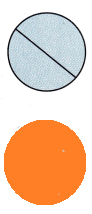Question 2.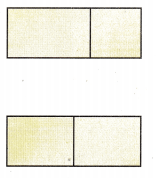Question 3.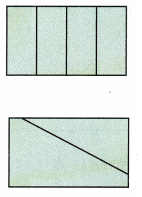Circle the shape that shows equal parts.

Question 4.Problem Solving

Write equal or unequal to describe the parts.

Question 5.
4 ___________ shares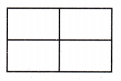Question 6.
4 ___________ shares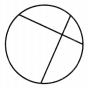Question 7.
2 ____________ sharesLesson Check

Question 8.
Ginger has a cheese sandwich. She will share the sandwich with her sister. Which sandwich shows 2 equal parts?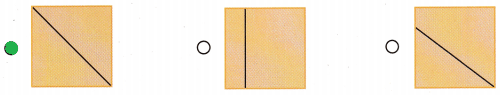Question 9.
Lucy has a pizza to share with 3 friends. She wants everyone to get an equal share. Which pizza shows 4 equal parts?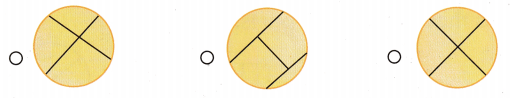Question 10.
Multi-Step Hank has a ball of dough. He wants to cut it into 2 equal parts. Then he wants to cut each part into 2 more equal parts. How many parts will Hank have?
(A) 2
(B) 4
(C) 8